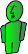# Instantaneous Release into the Air

The three dimensional nature of our atmosphere often makes tracking airborne pollutants very difficult. This section of Fate allows you to estimate the concentration of a pollutant at a specific distance downwind from an instantaneous release of pollutant. An example of a source that might release this type of pollutant puff is the explosion of an ammonia tank.

Step 1 Manually convert input data to metric units: meters, grams, and seconds.
Step 2: Enter conditions
Step 3: Choose atmospheric stability

A, B = Unstable
C, D = Neutral
E, F = Stable

Day Radiation Intensity Night Cloud Cover
Wind Speed (m/s) Strong Medium Slight Cloudy Calm and Clear
< 2 A A-B B
2-3 A-B B C E F
3-5 B B-C C D E
5-6 C C-D D D D
> 6 C D D D D
Step 4: Enter the dispersion coefficients

#### Vertical

Unstable:
Neutral:
Stable:
Unstable:
Neutral:
Stable:
Concentration calculations

Time since release: seconds

$T = \frac{x}{ū}$

Result: g/m3

Graph varying distance in Y
$C_{(x,y,z,t)} = \frac{Q_T}{(2\pi)^{\frac{3}{2}}\sigma_x\sigma_y\sigma_z}e^{(-\frac{1}{2}(\frac{y}{\sigma_y})^2)}(e^{(-\frac{1}{2}(\frac{z-H_r}{\sigma_z})^2)}+e^{(-\frac{1}{2}(\frac{z+H_r}{\sigma_z})^2)})$Graph varying distance in Z
$C_{(x,y,z,t)} = \frac{Q_T}{(2\pi)^{\frac{3}{2}}\sigma_x\sigma_y\sigma_z}e^{(-\frac{1}{2}(\frac{y}{\sigma_y})^2)}(e^{(-\frac{1}{2}(\frac{z-H_r}{\sigma_z})^2)}+e^{(-\frac{1}{2}(\frac{z+H_r}{\sigma_z})^2)})$